[译]Godot系列教程六 - 简单的二维游戏

+关注继续查看

Pong

Godot自带的Demo中有大量更复杂的示例，但这款叫“Pong”的游戏可以对2D游戏的基本特性做一个介绍。

场景设置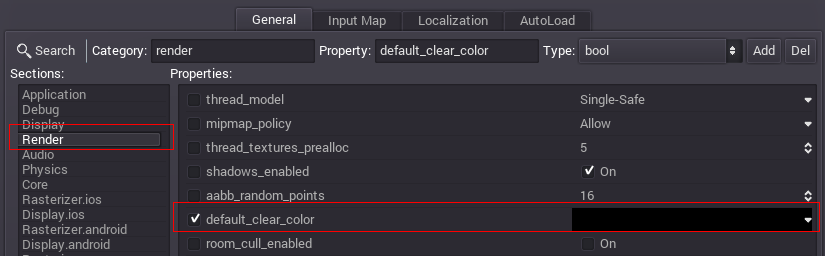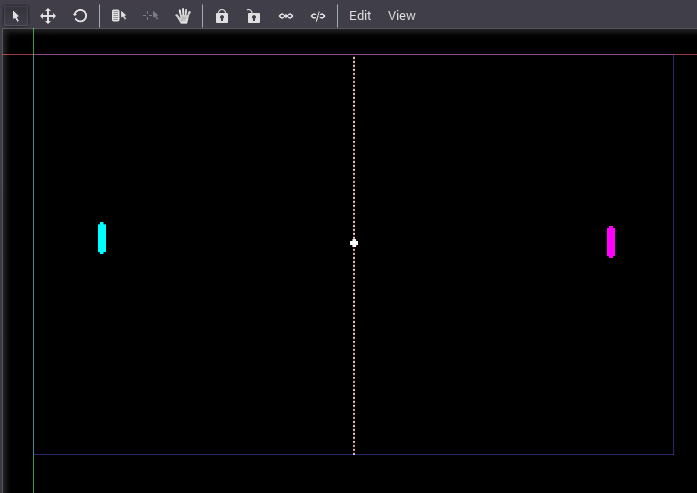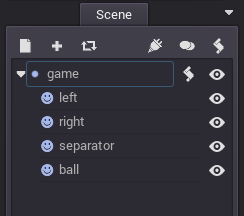输入动作设置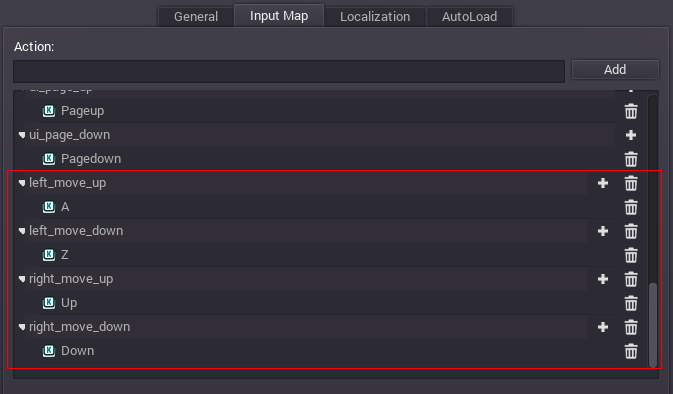脚本

extends Node2D

pass

extends Node2D

# 成员变量
var screen_size
var direction = Vector2(1.0, 0.0)

screen_size = get_viewport_rect().size
set_process(true)

# 成员变量
var screen_size
var direction = Vector2(1.0, 0.0)

# 常量，球初始移动速度（单位：像素/秒）
const INITIAL_BALL_SPEED = 80
# 球的实时速度（单位：像素/秒）
var ball_speed = INITIAL_BALL_SPEED

screen_size = get_viewport_rect().size
set_process(true)

func _process(delta):

var ball_pos = get_node("ball").get_pos()

ball_pos += direction * ball_speed * delta

if ( (ball_pos.y < 0 and direction.y < 0) or (ball_pos.y > screen_size.y and direction.y > 0)):
direction.y = -direction.y

if ( (left_rect.has_point(ball_pos) and direction.x < 0) or (right_rect.has_point(ball_pos) and direction.x > 0)):
direction.x = -direction.x
ball_speed *= 1.1
direction.y = randf() * 2.0 - 1
direction = direction.normalized()

if (ball_pos.x < 0 or ball_pos.x > screen_size.x):
ball_pos = screen_size * 0.5  # ball goes to screen center
ball_speed = 80
direction = Vector2(-1, 0)

get_node("ball").set_pos(ball_pos)

var left_pos = get_node("left").get_pos()

if (left_pos.y > 0 and Input.is_action_pressed("left_move_up")):
if (left_pos.y < screen_size.y and Input.is_action_pressed("left_move_down")):

get_node("left").set_pos(left_pos)

var right_pos = get_node("right").get_pos()

if (right_pos.y > 0 and Input.is_action_pressed("right_move_up")):
if (right_pos.y < screen_size.y and Input.is_action_pressed("right_move_down")):

get_node("right").set_pos(right_pos)[译]Godot系列教程六 - 简单的二维游戏
Pong Godot自带的Demo中有大量更复杂的示例，但这款叫“Pong”的游戏可以对2D游戏的基本特性做一个介绍。 静态资源 本文所用到的一些资源文件：http://files.cnblogs.com/files/x3d/pong_assets.zip 场景设置 考虑到兼容旧设备，该游戏的分辨率设置为 640x400像素，相关操作在项目设置中进行。
910 0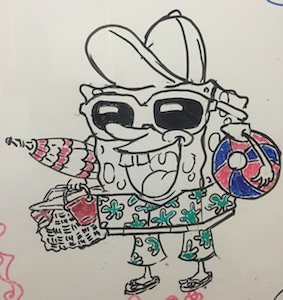DotNetNuke模块制作Super-Simple(DAL+)教程-翻译

947 01065 07251 0688 0[译]Godot系列教程一 - 场景与节点

923 08947 0907 0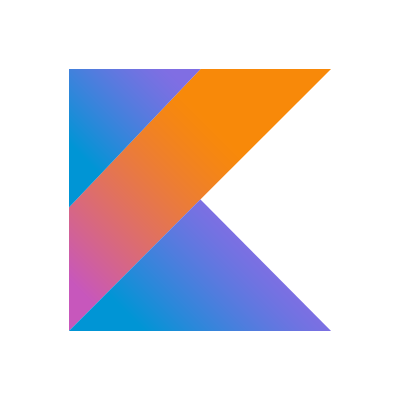974 0[译]Godot系列教程五 - 制作Godot编辑器插件

1116 0
x3d

527

0

《Nacos架构&原理》

《看见新力量：二》电子书